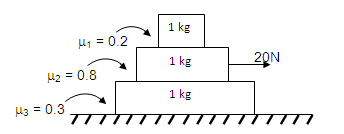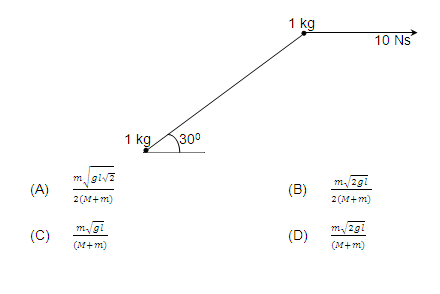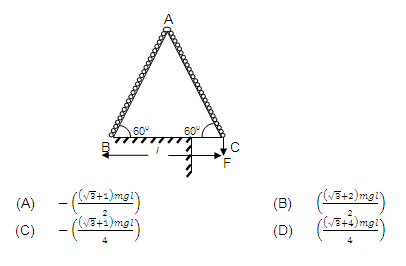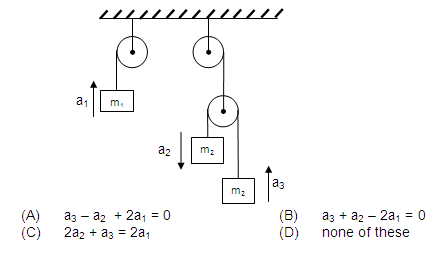Click to Chat

1800-1023-196

+91-120-4616500

CART 0

• 0

MY CART (5)

Use Coupon: CART20 and get 20% off on all online Study Material

ITEM
DETAILS
MRP
DISCOUNT
FINAL PRICE
Total Price: Rs.

There are no items in this cart.
Continue Shopping• Complete JEE Main/Advanced Course and Test Series
• OFFERED PRICE: Rs. 15,900
• View Details

```PHYSICS SECTION-I PART-A

Straight Objective Type

This section contains 9 multiple choice questions numbered 1 to 9. Each question has 4 choices (A), (B), (C) and (D) out of which ONLY ONE is correct.

1. Two blocks of masses 2 kg and 4 kg are connected through a massless inextensible string. The co-efficient of friction between 2 kg block and ground is 0.4 and the coefficient of friction between 4 kg block and ground is 0.6. Two forces F1 = 10 N and F2 = 20 N are applied on the blocks as shown in the figure. Calculate the frictional force between 4 kg block and ground (Assume initially the tension in the string was just zero before forces F1 and F2 were applied)(A) 24 N                                                         (B) 8 N
(C) 18 N                                                        (D) 10 N

2. Three large sides blocks are kept stationary over one another as shown in the figure. A horizontal force of 20 N is acting on the middle block as shown in the figure. Choose the incorrect statement.(A) The acceleration of lowest block will be 4.5 m/s2
(B) The acceleration of uppermost block will be 2 m/s2
(C) The frictional force between middle and lower block will be 12 N.
(D) The frictional force between middle and upper block will be 2N.

3. A particle of mass m kept at the origin is subjected to a force F--> = (pt – qx) i  where t is the time elapsed and x is the x co-ordinate of the position of the particle. Particle starts its motion at t = 0 with zero initial velocity. If p and q are positive constants, then:
(A) The acceleration of the particle will continuously keep on increasing with time.
(B) Particle will execute simple harmonic motion
(C) The force on the particle will have no upper limit.
(D) The acceleration of particle will vary sinusoidally with time.5. Consider the adjacent figure. The cube shaped carriage ABCDEFGH of side l has a mass M and it can slide over two frictionless rails PQ and RS. A shot of mass m is thrown from corner A such that it lands at corner F. The angle of projected as seen from the carriage is 450. While the shot is in the air, the velocity of carriage as seen from the ground is :6. Two balls of masses 1 kg each are connected by an inextensible massless string. The system is resting on a smooth horizontal surface. An impulse of 10 Ns is applied to one of balls at an angle 300 with the line joining two balls in horizontal direction as shown in the figure. Assuming that the string remains taut after the impulse, the magnitude of impulse of tension is:
(A) 6 Ns                                                     (B) 5/2 √3 Ns
(C) 5 Ns                                                    (D) 5/√3 Ns

7. A fixed wedge ABC is in the shape of an equilateral triangle of side l. Initially, a chain of length 2l and mass m rests on the wedge as shown. The chain is slowly being pulled down by the application of a force F as shown. Work done by gravity till the time, the chain leaves the wedge will be :8. In the adjacent figure, all the pulleys are frictionless and massless, all the strings are also massless. The relation between a1, a2 and a3 is (Where a1, a2 and a3 are the accelerations of masses m1, m2 and m3 respectively in the direction as shown in the figure)2 / Next >>

```### Course Features

• 731 Video Lectures
• Revision Notes
• Previous Year Papers
• Mind Map
• Study Planner
• NCERT Solutions
• Discussion Forum
• Test paper with Video Solution